Get instant live expert help with Excel or Google Sheets“My Excelchat expert helped me in less than 20 minutes, saving me what would have been 5 hours of work!”

#### Post your problem and you’ll get expert help in seconds.

Your message must be at least 40 characters
Our professional experts are available now. Your privacy is guaranteed.

# How to calculate a retirement date

Many companies have a policy that states employees have to retire after a certain age. We can calculate this retirement date from the birth date. To do this, we need to use the EDATE and YEARFRAC functions in Excel. In this tutorial, we will learn how to calculate retirement date from birth date.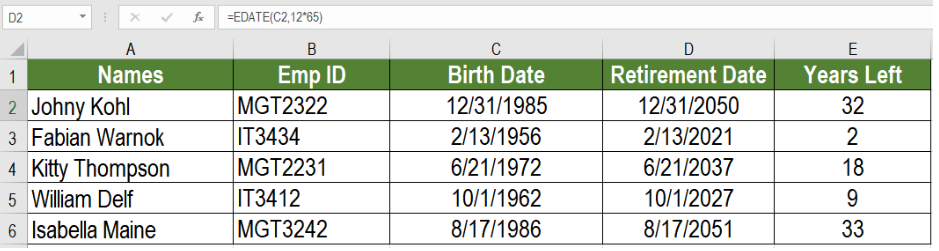Figure 1. Example of How to Calculate Retirement Date

## Generic Formula

`=EDATE (birth_date,12*retirement_age)`

## How this Formula Works

The EDATE function takes a date, adds a certain number of months to it and returns the result as a serial date. This formula takes the birth_date and retirement_age as the arguments for EDATE. To add the months with this date, we need to add the retirement age times 12 months.

The following data set contains an employee information data set. Column A, B and C has the employee names, IDs and birth dates.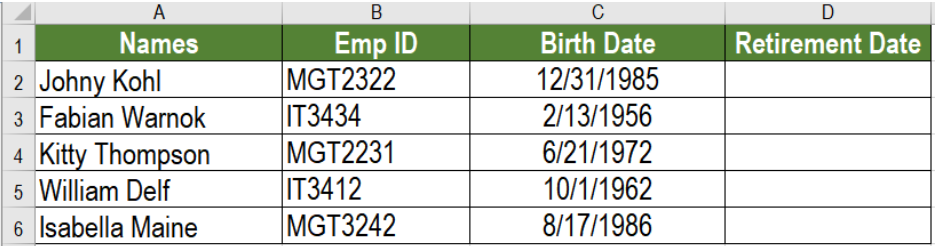Figure 2. The Sample Data Set

For this example, we will consider the retirement date to 65. To calculate the retirement dates in column D:

• We need to select cell D2.
• Assign the formula `=EDATE (C2,12*65) `to D2.
• Press Enter.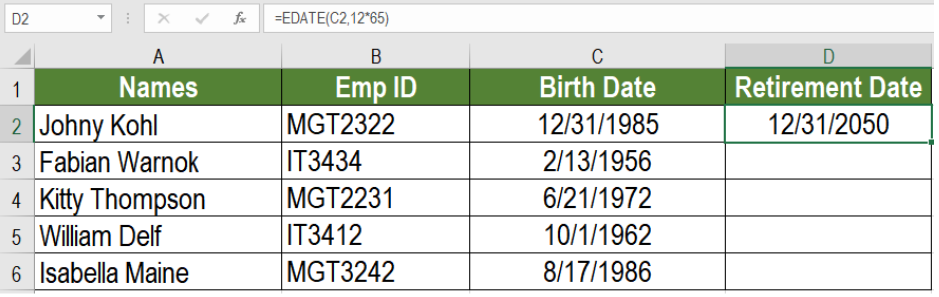Figure 3. Applying the Formula to the Data

This will show the retirement date in cell D2. Finally, dragging the formula from cells D2 to D6 will make column D show the dates.

## Calculating the Years Left till Retirement

We can also calculate the years remaining from the previous formula. To calculate the years remaining in Column E:

• Go to cell E2.
• Assign the formula  `=YEARFRAC(TODAY(),D2) `to cell E2.
• Press Enter to apply the formula to E2.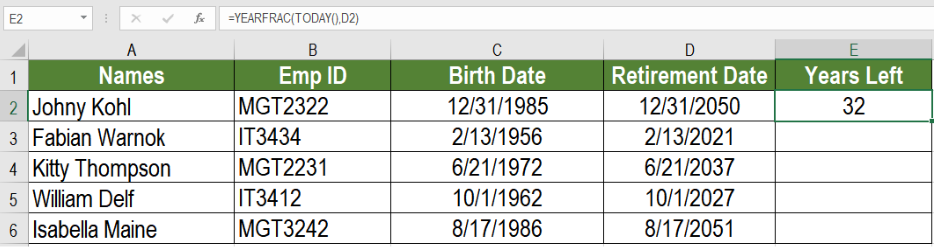Figure 4. Calculating the Years Remaining

Next, we need to drag the formula from cells E2 to E6. We can do this dragging the fill handle in the bottom right. Now, column E will show the years remaining to retire.

## Notes

• You can only find the year of retirement by slightly modifying the formula. To do that you can simply nest the previous formula in a YEAR function, =YEAR(EDATE(C2,12*65)).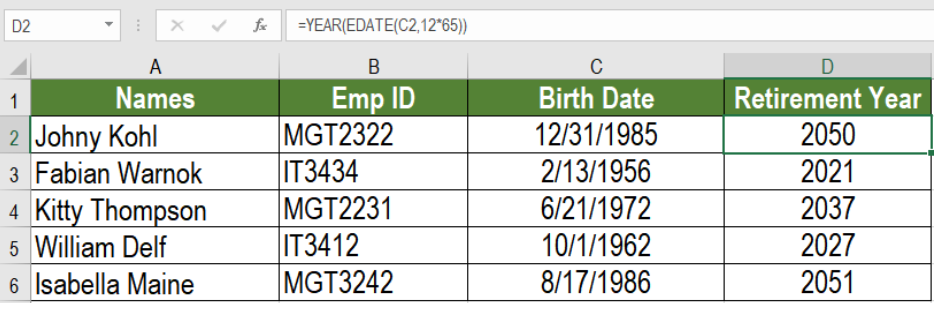Figure 5. Calculating the Retirement Date Only

Most of the time, the problem you will need to solve will be more complex than a simple application of a formula or function. If you want to save hours of research and frustration, try our live Excelchat service! Our Excel Experts are available 24/7 to answer any Excel question you may have. We guarantee a connection within 30 seconds and a customized solution within 20 minutes.

### Did this post not answer your question? Get a solution from connecting with the expert.Another blog reader asked this question today on Excelchat:
Solution examplesI need a formula that looks up if the date (which looks like "8/1/2018 8:05:36 AM") in Column "C" contains year 2018. And then populates "2018" in Column D, if the date does not contain "2018" then enter False in Column D.
Solved by F. H. in 20 minscan you teach me the steps to create a nested if function, and to nest an AND function inside of an IF function?
Solved by A. Q. in 22 minsa date formula that results in showing the date as 29/12/00 (date format as dd/mm/yy) - use column BA to calculate 364 days (same date format) in column BS, if BA blank, then calculate 364 days using column AX. Results for column BA are correct. However, if column BA is empty, then it should calculate using column AX, which has data, but the result is always 29/12/00, regardless of the date in column AX. I have used this formula with success in another workbook, but this file wont work! Formula: =IF(AND(LEN(\$BA74)=0,LEN(\$AX74)=0),"",IF(LEN(\$BA74)=0,DATE(YEAR(\$AX74),MONTH(\$AX74),DAY(\$AX74)+364),DATE(YEAR(\$BA74),MONTH(\$BA74),DAY(\$BA74)+364)))
Solved by A. L. in 60 minsneed help in this =IF(C2="","",IF(ISBLANK(IF(C2="yes",HLOOKUP(VALUE(MONTH(A2) & "/" & DAY(A2) & "/" & YEAR(A2)),Sheet2!\$B\$1:\$AA\$1000,2,FALSE),IF(C2="no",HLOOKUP(VALUE(MONTH(A2) & "/" & DAY(A2) & "/" & YEAR(A2)),Sheet2!\$B\$1:\$AA\$1000,3,FALSE),""))),"NOT YET",IF(C2="yes",HLOOKUP(VALUE(MONTH(A2) & "/" & DAY(A2) & "/" & YEAR(A2)),Sheet2!\$B\$1:\$AA\$1000,2,FALSE),IF(C2="no",HLOOKUP(VALUE(MONTH(A2) & "/" & DAY(A2) & "/" & YEAR(A2)),Sheet2!\$B\$1:\$AA\$1000,3,FALSE),""))))
Solved by V. Q. in 11 minsneed help =IF(C2="","",IF(ISBLANK(IF(C2="yes",HLOOKUP(VALUE(MONTH(A2) & "/" & DAY(A2) & "/" & YEAR(A2)),Sheet2!\$B\$1:\$AA\$1000,2,FALSE),IF(C2="no",HLOOKUP(VALUE(MONTH(A2) & "/" & DAY(A2) & "/" & YEAR(A2)),Sheet2!\$B\$1:\$AA\$1000,3,FALSE),""))),"NOT YET",IF(C2="yes",HLOOKUP(VALUE(MONTH(A2) & "/" & DAY(A2) & "/" & YEAR(A2)),Sheet2!\$B\$1:\$AA\$1000,2,FALSE),IF(C2="no",HLOOKUP(VALUE(MONTH(A2) & "/" & DAY(A2) & "/" & YEAR(A2)),Sheet2!\$B\$1:\$AA\$1000,3,FALSE),""))))
Solved by G. S. in 9 mins## Subscribe to Excelchat.coAnother blog reader asked this question today on Excelchat: